﻿ 低渗–致密气藏压裂井产能理论计算方法 Study on Productivity Calculation Model of Fractured Well in Low Permeability-Tight Gas Reservoir

Vol.08 No.02(2018), Article ID:25593,8 pages
10.12677/APF.2018.82005

Study on Productivity Calculation Model of Fractured Well in Low Permeability-Tight Gas Reservoir

Shihua Liu*, Tingxue Jiang, Chunfang Wu, Kuiwei Li

SINOPEC Research Institute of Petroleum Engineering, Beijing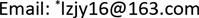Received: Jun. 5th, 2018; accepted: Jun. 20th, 2018; published: Jun. 27th, 2018ABSTRACT

With the development of more and more low-permeability tight gas reservoir, hydraulic fracturing is needed due to the low natural productivity. Productivity of low permeability fractured well has been studied by many scholars, however, the calculation result is usually large while the influencing factors of productivity are not simultaneously considered, including the stress sensitivity, threshold pressure gradient with gas flow and high-speed non-Darcy effect of hydraulic fracture near the well. In this paper, on the basis of disturbing ellipse and rectangular family, the productivity calculation formula of fractured well in low permeability tight gas reservoir is derived theoretically with consideration of more factors and the corresponding productivity curve is plotted. Result shows that, the influencing factors of fractured well productivity in low permeability tight gas reservoir include threshold pressure gradient, deformed media coefficient, high-speed non-Darcy flow factor and fracture parameters. Single factor sensitivity analysis plate is also protracted, which has important theoretical value and practical meaning.

Keywords:Low Permeability-Tight Gas Reservoir, Fracturing, Threshold Pressure Gradient, High-Speed Non-Darcy Flow, Stress Sensitivity1. 引言

2. 低渗致密气藏复杂渗流机理表征方法

$v=\frac{k}{{\mu }_{g}}\left(\frac{\partial p}{\partial y}-G\right)$ (1)

$\frac{\text{d}p}{\text{d}x}=\frac{\mu }{k}v+\beta {\rho }_{g}{v}^{2}$ (2)

$v=\frac{{q}_{m}}{{\rho }_{g}A}=\frac{{\rho }_{gsc}{q}_{sc}}{{\rho }_{g}{w}_{f}h}$ (3)

${\rho }_{gsc}=\frac{{p}_{sc}M}{R{T}_{sc}{Z}_{sc}}$ (4)

${\rho }_{g}=\frac{pM}{RTZ}$ (5)

$v=\frac{1}{{w}_{f}h}\frac{{p}_{sc}{q}_{sc}TZ}{{T}_{sc}{Z}_{sc}p}$ (6)

$\frac{\text{d}p}{\text{d}x}=\frac{\mu }{{k}_{f}{w}_{f}h}\frac{{p}_{sc}{q}_{sc}TZ}{{T}_{sc}{Z}_{sc}p}+\frac{\beta M}{{w}_{f}^{2}{h}^{2}TZp}{\left[\frac{{p}_{sc}{q}_{sc}TZ}{{T}_{sc}{Z}_{sc}}\right]}^{2}$ (7)

${k}_{f}={k}_{fi}{\text{e}}^{-{\alpha }_{f}\left({p}_{i}-p\right)}$ (8)

$\frac{\text{d}p}{\text{d}x}=\frac{\mu }{{k}_{fi}{\text{e}}^{-{\alpha }_{f}\left({p}_{i}-p\right)}{w}_{f}h}\frac{{p}_{sc}{q}_{sc}TZ}{{T}_{sc}{Z}_{sc}p}+\frac{\beta M}{{w}_{f}^{2}{h}^{2}TZp}{\left[\frac{{p}_{sc}{q}_{sc}TZ}{{T}_{sc}{Z}_{sc}}\right]}^{2}$ (9)

$b=\frac{{\text{e}}^{{\alpha }_{f}{p}_{i}}\mu }{{k}_{fi}{w}_{f}h}\frac{{p}_{sc}{q}_{sc}TZ}{{T}_{sc}{Z}_{sc}}$$c=\frac{\beta M}{{w}_{f}^{2}{h}^{2}TZ}{\left[\frac{{p}_{sc}{q}_{sc}TZ}{{T}_{sc}{Z}_{sc}}\right]}^{2}$

$\frac{p{\text{e}}^{{\alpha }_{f}p}}{b+c{\text{e}}^{{\alpha }_{f}p}}\text{d}p=\text{d}x$ (10)

$x=\frac{1}{{\alpha }_{f}^{2}c}\left[di\mathrm{log}\left(1+\frac{c}{b}{\text{e}}^{{\alpha }_{f}p}\right)-di\mathrm{log}\left(1+\frac{c}{b}{\text{e}}^{{\alpha }_{f}{p}_{wf}}\right)\right]+\frac{p}{{\alpha }_{f}c}\mathrm{ln}\frac{b+c{\text{e}}^{{\alpha }_{f}p}}{b+c{\text{e}}^{{\alpha }_{f}{p}_{wf}}}$ (11)

${x}_{f}=\frac{1}{{\alpha }_{f}^{2}c}\left[di\mathrm{log}\left(1+\frac{c}{b}{\text{e}}^{{\alpha }_{f}{p}_{f}}\right)-di\mathrm{log}\left(1+\frac{c}{b}{\text{e}}^{{\alpha }_{f}{p}_{wf}}\right)\right]+\frac{{p}_{f}}{{\alpha }_{f}c}\mathrm{ln}\frac{b+c{\text{e}}^{{\alpha }_{f}{p}_{f}}}{b+c{\text{e}}^{{\alpha }_{f}{p}_{wf}}}$ (12)

3. 压裂裂缝激发的椭圆渗流

$\stackrel{¯}{x}={x}_{f}ch\left(\xi \right)$ (13)

$\stackrel{¯}{y}=\frac{2}{\text{π}}{\int }_{0}^{\frac{\text{π}}{2}}b\mathrm{sin}\left(\eta \right)\text{d}\eta =\frac{2{x}_{f}}{\text{π}}sh\left(\xi \right)$ (14)

$v=\frac{k}{{\mu }_{g}}\left(\frac{\partial p}{\partial \stackrel{¯}{y}}-G\right)$ (15)

${\rho }_{v}=\rho \frac{k}{{\mu }_{g}}\left(\frac{\partial p}{\partial \stackrel{¯}{y}}-G\right)=\frac{{\rho }_{sc}{q}_{sc}}{4{x}_{f}hch\left(\xi \right)}$ (16)

$\frac{\text{d}p}{\text{d}\stackrel{¯}{y}}=\frac{\text{d}p}{\text{d}\xi }\frac{\text{d}\xi }{\text{d}\stackrel{¯}{y}}=\frac{\text{d}p}{\text{d}\xi }\frac{\text{π}}{2{x}_{f}}\frac{1}{ch\left(\xi \right)}$ (17)

$k\frac{p}{{\mu }_{g}Z}\left[\frac{\text{d}p}{\text{d}\xi }\frac{\text{π}}{2{x}_{f}}\frac{1}{ch\left(\xi \right)}-G\right]=\frac{{p}_{sc}T}{{T}_{sc}{Z}_{sc}}\frac{{q}_{sc}}{4{x}_{f}hch\left(\xi \right)}$ (18)

$k{\text{e}}^{-{\alpha }_{m}\left({p}_{i}-p\right)}\frac{p}{{\mu }_{g}Z}\left[\frac{\text{d}p}{\text{d}\xi }\frac{\text{π}}{2{x}_{f}}\frac{1}{ch\left(\xi \right)}-G\right]=\frac{{p}_{sc}T}{{T}_{sc}{Z}_{sc}}\frac{{q}_{sc}}{4{x}_{f}hch\left(\xi \right)}$ (19)

$p{\text{e}}^{{\alpha }_{m}p}\left[\frac{\text{d}p}{\text{d}\xi }-2{x}_{f}ch\left(\xi \right)G/\text{π}\right]=\frac{{p}_{sc}{\text{e}}^{{\alpha }_{m}p}{\mu }_{g}ZT}{k{T}_{sc}{Z}_{sc}}\frac{{q}_{sc}}{2\text{π}}$ (20)

$A=2{x}_{f}G/\text{π},\text{\hspace{0.17em}}B=\frac{{p}_{sc}{\text{e}}^{{\alpha }_{m}p}{\mu }_{g}ZT}{k{T}_{sc}{Z}_{sc}}\frac{{q}_{sc}}{2\text{π}}$

$p{\text{e}}^{{\alpha }_{m}p}\left[\frac{\text{d}p}{\text{d}\xi }-Ach\left(\xi \right)\right]=B$ (21)

${\xi }_{e}=\mathrm{ln}\frac{2{r}_{e}}{{x}_{f}},\text{\hspace{0.17em}}p={p}_{e}$

$\begin{array}{l}A\frac{{x}_{f}}{{r}_{e}}-AB\frac{{x}_{f}}{{r}_{e}}\frac{1+{\alpha }_{m}{p}_{e}}{{p}_{e}^{2}{\text{e}}^{\alpha {p}_{e}}}-AB\frac{{x}_{f}}{{r}_{e}}\frac{2+{\alpha }_{m}^{2}{p}_{e}^{2}}{{p}_{e}^{5}{\text{e}}^{{\alpha }_{m}{p}_{e}}}\left(\frac{B}{{p}_{e}{\text{e}}^{{\alpha }_{m}{p}_{e}}}+A\frac{{x}_{f}}{{r}_{e}}\right)+\left(-{B}^{2}\frac{1+{\alpha }_{m}{p}_{e}}{{p}_{e}^{3}{\text{e}}^{2{\alpha }_{m}{p}_{e}}}\\ -AB\frac{{x}_{f}}{{r}_{e}}\frac{1+{\alpha }_{m}{p}_{e}}{{p}_{e}^{2}{\text{e}}^{{\alpha }_{m}{p}_{e}}}+A\frac{{x}_{f}}{{r}_{e}}\right)\left[{B}^{2}\frac{2{\alpha }_{m}^{2}{p}_{e}+4{\alpha }_{m}+3}{{p}_{e}^{3}{\text{e}}^{2{\alpha }_{m}{p}_{e}}}+AB\frac{{x}_{f}}{{r}_{e}}\left({\alpha }_{m}^{2}{p}_{e}^{2}+2{\alpha }_{m}{p}_{e}+2\right)\right]\\ +\left(\frac{B}{{p}_{e}{\text{e}}^{{\alpha }_{m}{p}_{e}}}+A\frac{{x}_{f}}{{r}_{e}}\right)\left[AB\frac{{x}_{f}}{{r}_{e}}\left({\alpha }_{m}^{2}{p}_{e}^{2}+2{\alpha }_{m}{p}_{e}+2\right)-\frac{4{\alpha }_{m}^{3}{p}_{e}^{2}+\left(12{\alpha }_{m}^{2}+6{\alpha }_{m}\right){p}_{e}+12{\alpha }_{m}+9}{{p}_{e}^{4}{\text{e}}^{2{\alpha }_{m}{p}_{e}}}\right]\\ +AB\frac{{x}_{f}}{{r}_{e}}\left(2{\alpha }_{m}^{2}{p}_{e}+2{\alpha }_{m}\right){\left(\frac{B}{{p}_{e}{\text{e}}^{{\alpha }_{m}{p}_{e}}}+A\frac{{x}_{f}}{{r}_{e}}\right)}^{2}-\left(-{B}^{2}\frac{1+{\alpha }_{m}{p}_{u}}{{p}_{u}^{3}{\text{e}}^{2{\alpha }_{m}p}}-AB\frac{1+{\alpha }_{m}{p}_{u}}{{p}_{u}^{2}{\text{e}}^{{\alpha }_{m}{p}_{u}}}\right)\end{array}$

$\begin{array}{l}×\left[{B}^{2}\frac{2{\alpha }_{m}^{2}{p}_{u}+4{\alpha }_{m}+3}{{p}_{u}^{3}{\text{e}}^{2{\alpha }_{m}{p}_{u}}}+AB\left({\alpha }_{m}^{2}{p}_{u}^{2}+2{\alpha }_{m}{p}_{u}+2\right)\right]\\ -\left(\frac{B}{{p}_{u}{\text{e}}^{{\alpha }_{m}{p}_{u}}}\right)\left[-\frac{4{\alpha }_{m}^{3}{p}_{u}^{2}+\left(12{\alpha }_{m}^{2}+6{\alpha }_{m}\right){p}_{u}+12{\alpha }_{m}+9}{{p}_{u}^{4}{\text{e}}^{2{\alpha }_{m}{p}_{u}}}\right]\\ +AB\frac{1+{\alpha }_{m}{p}_{u}}{{p}_{u}^{2}{\text{e}}^{{\alpha }_{m}{p}_{u}}}-AB\left(2{\alpha }^{2}{p}_{u}+2{\alpha }_{m}\right){\left(\frac{B}{{p}_{e}{\text{e}}^{{\alpha }_{m}{p}_{u}}}+A\right)}^{2}=0\end{array}$ (22)

4. 低渗致密气藏压裂井实例计算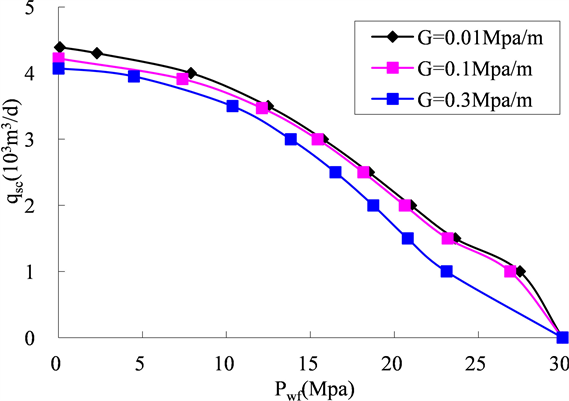Figure 1. Influence law of threshold pressure gradient on productivity change in low permeability tight gas reservoir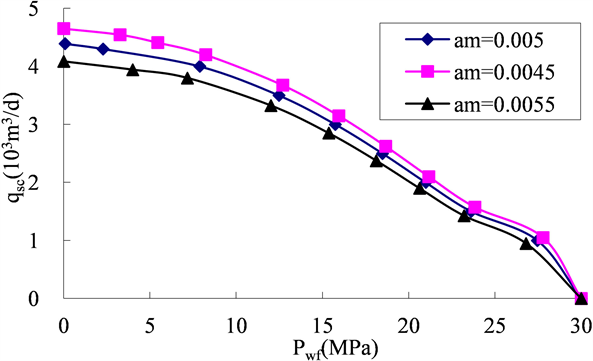Figure 2. Influence law of deformed media on productivity change in low permeability tight gas reservoir

5. 结论

1) 基于扰动椭圆及发展的矩形族思想，给出了同时考虑启动压力梯度、裂缝近井地带高速非达西效应、气藏基质及裂缝应力敏感性等因素影响的压裂井产能计算方法，并给出了解析解。

2) 拟启动压力梯度、高速非达西渗流系数、压裂变形介质系数、裂缝长度以及裂缝导流能力均对产能有影响。保持井底压力不变前提下，拟启动压力梯度、高速非达西渗流系数、介质变形系数越小，压裂气井产能越大；裂缝长度越长、裂缝导流能力越大，压裂气井产能越大。同时，随着井底流压升高，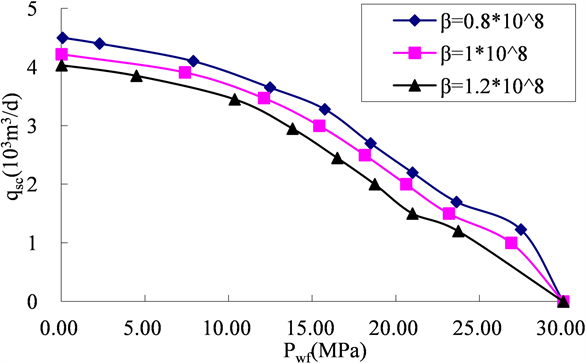Figure 3. Influence law of high speed non-Darcy flow coefficient on productivity change in low permeability tight gas reservoir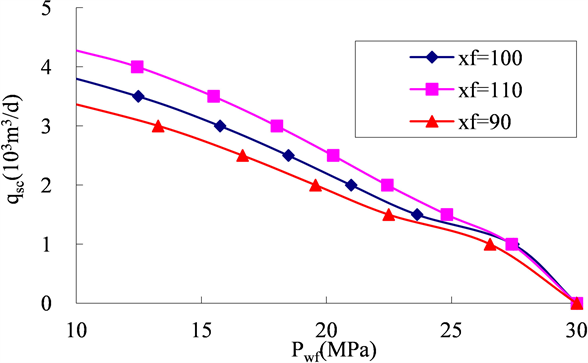Figure 4. Influence law of fracture length on productivity change in low permeability tight gas reservoir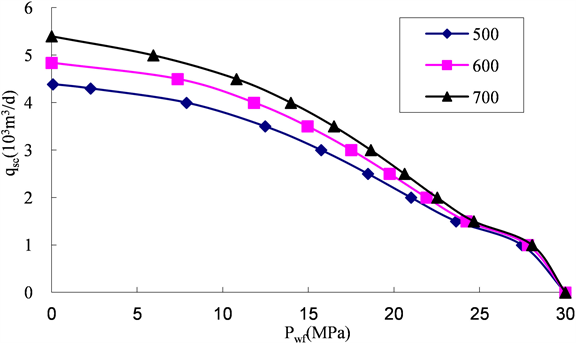Figure 5. Influence law of fracture conductivity on productivity change in low permeability tight gas reservoir

3) 对于低渗致密气藏，在对压裂气井进行配产设计时一定要考虑拟启动压力梯度、高速非达西效应以及介质变形系数对产能的影响，同时在压裂设计时要优化裂缝的长度及导流能力。

Study on Productivity Calculation Model of Fractured Well in Low Permeability-Tight Gas Reservoir[J]. 渗流力学进展, 2018, 08(02): 35-42. https://doi.org/10.12677/APF.2018.82005

1. 1. 雷群, 杨正明, 刘先贵, 等. 复杂天然气藏储层特征及渗流规律[M]. 北京: 石油工业出版社, 2008: 48-53.

2. 2. 罗天雨, 赵金洲, 郭建春. 求取压裂后气井产能的椭圆流方法[J]. 天然气工业, 2005, 25(10): 94-96.

3. 3. 汪永利, 蒋廷学, 曾斌. 气井压裂后稳态产能的计算[J]. 石油学报, 2003, 24(4): 65-68.

4. 4. 杨正明, 张松, 张训华, 等. 气井压后稳态产能公式和压裂数值模拟研究[J]. 天然气工业, 2003, 23(4): 74-76.

5. 5. 周琦, 姜汉桥, 李志刚. 低渗透气藏垂直裂缝井产能计算[J]. 油气井测试, 2009, 18(2): 8-11.

6. 6. 雷刚, 董春涛, 杨书等. 致密砂岩气藏垂直压裂井拟稳态流动产能分析[J]. 大庆石油地质与开发, 2014, 33(1): 170-174.

7. 7. 邓英尔, 刘慈群, 王允诚. 垂直裂缝井两相非达西椭圆渗流特征线解,差分解及开发指标计算方法[J]. 石油勘探与开发, 2000, 27(1): 60-63.

8. 8. Rushing, J.A., Newsham, K.E., Lasswell, P.M., et al. (2004) Klinkenberg-Corrected Permeability Measurements in Tight Gas Sands: Steady-State versus Unsteady-State Techniques. SPE89867, 1-11.

9. 9. 梁冰, 姜云, 孙维吉. 低渗气藏滑脱效应对启动压力梯度的影响试验研究[J]. 渗流力学进展, 2018, 8(2): 15-21. https://doi.org/10.12677/APF.2018.82003

10. 10. Ruth, D. and Ma, H.P. (1992) On the Derivation of the Forchheimer Equation by Means of the Average Theorem. Transport in Porous Media, 7, 255-264. https://doi.org/10.1007/BF01063962

11. 11. Barree, R.D. and Conway, M.W. (2004) Beyond Beta Factors: A Complete Model for Darcy Forchheimer and Trans-Forchheimer Flow in Porous Media. SPE Annual Technical Conference and Exhibition, Houston, 26-29 September, SPE89325.